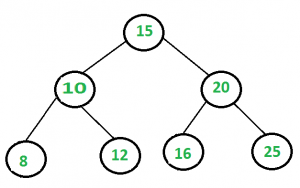Related Articles

# Sum of all nodes in a binary tree

• Difficulty Level : Easy
• Last Updated : 03 Sep, 2021

Give an algorithm for finding the sum of all elements in a binary tree.In the above binary tree sum = 106.

The idea is to recursively, call left subtree sum, right subtree sum and add their values to current node’s data.

## C++

 `/* Program to print sum of all the elements of a binary tree */``#include ``using` `namespace` `std;` `struct` `Node {``    ``int` `key;``    ``Node* left, *right;``};` `/* utility that allocates a new Node with the given key  */``Node* newNode(``int` `key)``{``    ``Node* node = ``new` `Node;``    ``node->key = key;``    ``node->left = node->right = NULL;``    ``return` `(node);``}` `/* Function to find sum of all the elements*/``int` `addBT(Node* root)``{``    ``if` `(root == NULL)``        ``return` `0;``    ``return` `(root->key + addBT(root->left) + addBT(root->right));``}` `/* Driver program to test above functions*/``int` `main()``{``    ``Node* root = newNode(1);``    ``root->left = newNode(2);``    ``root->right = newNode(3);``    ``root->left->left = newNode(4);``    ``root->left->right = newNode(5);``    ``root->right->left = newNode(6);``    ``root->right->right = newNode(7);``    ``root->right->left->right = newNode(8);` `    ``int` `sum = addBT(root);``    ``cout << ``"Sum of all the elements is: "` `<< sum << endl;` `    ``return` `0;``}`

## Java

 `// Java Program to print sum of``// all the elements of a binary tree``class` `GFG``{``static` `class` `Node``{``    ``int` `key;``    ``Node left, right;``}` `/* utility that allocates a new``   ``Node with the given key */``static` `Node newNode(``int` `key)``{``    ``Node node = ``new` `Node();``    ``node.key = key;``    ``node.left = node.right = ``null``;``    ``return` `(node);``}` `/* Function to find sum``   ``of all the elements*/``static` `int` `addBT(Node root)``{``    ``if` `(root == ``null``)``        ``return` `0``;``    ``return` `(root.key + addBT(root.left) +``                       ``addBT(root.right));``}` `// Driver Code``public` `static` `void` `main(String args[])``{``    ``Node root = newNode(``1``);``    ``root.left = newNode(``2``);``    ``root.right = newNode(``3``);``    ``root.left.left = newNode(``4``);``    ``root.left.right = newNode(``5``);``    ``root.right.left = newNode(``6``);``    ``root.right.right = newNode(``7``);``    ``root.right.left.right = newNode(``8``);` `    ``int` `sum = addBT(root);``    ``System.out.println(``"Sum of all the elements is: "` `+ sum);``}``}` `// This code is contributed by Arnab Kundu`

## Python3

 `# Python3 Program to print sum of all``# the elements of a binary tree` `# Binary Tree Node` `""" utility that allocates a new Node``with the given key """``class` `newNode:` `    ``# Construct to create a new node``    ``def` `__init__(``self``, key):``        ``self``.key ``=` `key``        ``self``.left ``=` `None``        ``self``.right ``=` `None``        ` `# Function to find sum of all the element``def` `addBT(root):``    ``if` `(root ``=``=` `None``):``        ``return` `0``    ``return` `(root.key ``+` `addBT(root.left) ``+``                       ``addBT(root.right))` `# Driver Code``if` `__name__ ``=``=` `'__main__'``:``    ``root ``=` `newNode(``1``)``    ``root.left ``=` `newNode(``2``)``    ``root.right ``=` `newNode(``3``)``    ``root.left.left ``=` `newNode(``4``)``    ``root.left.right ``=` `newNode(``5``)``    ``root.right.left ``=` `newNode(``6``)``    ``root.right.right ``=` `newNode(``7``)``    ``root.right.left.right ``=` `newNode(``8``)` `    ``sum` `=` `addBT(root)` `    ``print``(``"Sum of all the nodes is:"``, ``sum``)` `# This code is contributed by``# Shubham Singh(SHUBHAMSINGH10)`

## C#

 `using` `System;` `// C# Program to print sum of``// all the elements of a binary tree``public` `class` `GFG``{``public` `class` `Node``{``    ``public` `int` `key;``    ``public` `Node left, right;``}` `/* utility that allocates a new ``   ``Node with the given key */``public` `static` `Node newNode(``int` `key)``{``    ``Node node = ``new` `Node();``    ``node.key = key;``    ``node.left = node.right = ``null``;``    ``return` `(node);``}` `/* Function to find sum ``   ``of all the elements*/``public` `static` `int` `addBT(Node root)``{``    ``if` `(root == ``null``)``    ``{``        ``return` `0;``    ``}``    ``return` `(root.key + addBT(root.left) + addBT(root.right));``}` `// Driver Code``public` `static` `void` `Main(``string``[] args)``{``    ``Node root = newNode(1);``    ``root.left = newNode(2);``    ``root.right = newNode(3);``    ``root.left.left = newNode(4);``    ``root.left.right = newNode(5);``    ``root.right.left = newNode(6);``    ``root.right.right = newNode(7);``    ``root.right.left.right = newNode(8);` `    ``int` `sum = addBT(root);``    ``Console.WriteLine(``"Sum of all the elements is: "` `+ sum);``}``}` `// This code is contributed by Shrikant13`

## Javascript

 ``
Output

`Sum of all the elements is: 36`

Method 2 – Another way to solve this problem is by using Level Order Traversal. Every time when a Node is deleted from the queue, add it to the sum variable.

## C++

 `#include ``#include ``using` `namespace` `std;` `struct` `Node {``    ``int` `key;``    ``struct` `Node *left, *right;``};` `// Utility function to create a new node``Node* newNode(``int` `key)``{``    ``Node* temp = ``new` `Node;``    ``temp->key = key;``    ``temp->left = temp->right = NULL;``    ``return` `(temp);``}` `/*Function to find sum of all elements*/``int` `sumBT(Node* root)``{``      ``//sum variable to track the sum of``      ``//all variables.``    ``int` `sum = 0;``  ` `    ``queue q;` `      ``//Pushing the first level.``    ``q.push(root);` `      ``//Pushing elements at each level from``      ``//the tree.``    ``while` `(!q.empty()) {``        ``Node* temp = q.front();``        ``q.pop();``      ` `          ``//After popping each element from queue``          ``//add its data to the sum variable.``        ``sum += temp->key;` `        ``if` `(temp->left) {``            ``q.push(temp->left);``        ``}``        ``if` `(temp->right) {``            ``q.push(temp->right);``        ``}``    ``}``    ``return` `sum;``}` `// Driver program``int` `main()``{``    ``// Let us create Binary Tree shown in above example``    ``Node* root = newNode(1);``    ``root->left = newNode(2);``    ``root->right = newNode(3);``    ``root->left->left = newNode(4);``    ``root->left->right = newNode(5);``    ``root->right->left = newNode(6);``    ``root->right->right = newNode(7);``    ``root->right->left->right = newNode(8);` `    ``cout << ``"Sum of all elements in the binary tree is: "``         ``<< sumBT(root);``}` `//This code is contributed by Sarthak Delori`
Output
`Sum of all elements in the binary tree is: 36`

Time Complexity: O(n)
Auxiliary Space: O(n)

This article is contributed by Prakriti Gupta. If you like GeeksforGeeks and would like to contribute, you can also write an article using write.geeksforgeeks.org or mail your article to review-team@geeksforgeeks.org. See your article appearing on the GeeksforGeeks main page and help other Geeks.